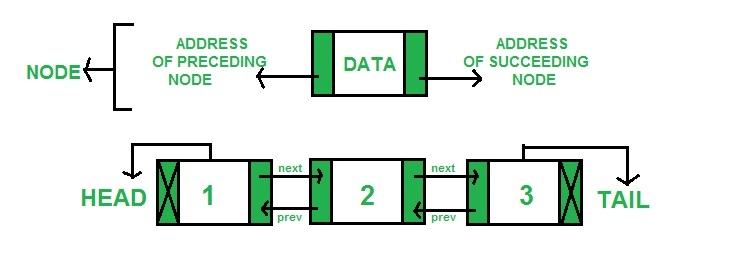dllist class of llist module in Python

• Last Updated : 05 Sep, 2020

llist is an extension module of CPython that provides a basic linked list structure. They are significantly faster that dequeue and even the standard list for that matter.

It is a type of linked list in each node stores data as well as two addresses (address of nodes succeeding and preceding it). A much simpler definition would be that in a doubly-linked list each node points to the node before it and also the node that comes immediately after it.
The following diagram explains it better:In llist, there is a dllist object that helps implement a doubly-linked list successfully.

Dllist Objects

class llist.dllist([iterable])
returns a new doubly linked list initialized from the iterables provided. If no iterables are given the linked list is produced but empty.

 import llist from llist import dllist    lst = llist.dllist(['first', 'second', 'third']) print(lst)

Output:

dllist([first, second, third])

dllist supports the following attributes:

• first : read only attribute, prints the first attribute of the list and None if the list is empty

 print(lst.first)

Output:

dllistnode(first)
• last : read only property, returns the last element of the list(tail) and None if the list is empty.

 print(lst.last)

Output:

dllistnode(third)
• size : read only attribute that returns the size of the list

 print(lst.size)

Output:

3

dllist also supports the following methods:

• append(x) : adds x to the right side of the list and returns a inserted dllist node. If x already is a dlist node then a new node is created and initialized with the value extracted from x.

 lst.append('fourth') print(lst)

Output:

dllist([first, second, third, fourth])
• appendleft(x) : adds x to the left side of the list and returns a inserted dllist node. If x already is a dllist node then a new node is created and initialized with the value extracted from x.

 lst.appendleft('fourth') print(lst)

Output:

dllist([fourth, first, second, third])
• appendright(x) : adds x to the right side of the list and returns a inserted dllist node. If x already is a dllist node then a new node is created and initialized with the value extracted from x.

 lst.appendright('fourth') print(lst)

Output:

dllist([first, second, third, fourth])
• clear() : removes all nodes from the list

 lst.clear() print(lst)

Output:

dllist()
• extend([iterable]) : adds elements from the iterable to the right side of the list.

 lst.extend(['fourth', 'fifth']) print(lst)

Output:

dllist([first, second, third, fourth, fifth])
• extendleft([iterable]) : adds elements from the iterable to the left side of the list

 lst.extendleft(['fourth', 'fifth']) print(lst)

Output:

dllist([fifth, fourth, first, second, third])
• extendright([iterable]) : adds elements from the iterable to the right side of the list

 lst.extendright(['fourth', 'fifth']) print(lst)

Output:

dllist([first, second, third, fourth, fifth])
• insert() : adds provided element to the right side of the list. It is usually used to insert element at any point in the list and for that the element it should be inserted before should be provided.

 lst.insert('fourth') node = lst.nodeat(2) lst.insert('fifth', node) print(lst)

Output:

dllist([first, second, fifth, third, fourth])
• nodeat(index) : returns node at a the specified index. Negative addresses are allowed.

 print(lst.nodeat(2)) print(lst.nodeat(-2))

Output:

dllistnode(third)
dllistnode(second)
• pop() : removes and returns an element’s value from the right side of the list.

 lst.pop() print(lst)

Output:

dllist([first, second])
• popleft() : removes and returns an element’s value from the left side of the list.

 lst.popleft() print(lst)

Output:

dllist([second, third])
• popright : removes and returns an element’s value from the right side of the list.

 lst.popright() print(lst)

Output:

dllist([first, second])
• remove() : removes the specified node form the list and returns the element stored in it.

 node = lst.nodeat(1) lst.remove(node) print(lst)

Output:

dllist([first, third])
• rotate(n) : if n is positive rotates the list n steps to the right but if it is negative rotate it n steps to the left

 lst.rotate(4) print(lst)

Output:

dllist([third, first, second])

In addition to these methods, dllist supports iteration, cmp(lst1, lst2), rich comparison operators, constant time len(lst), hash(lst), and subscript references lst for accessing elements by index.

Let’s further discuss more objects of llist that are related to dllist:

1) dllistnode

Implements a node in a doubly linked list which can optionally be initialized if value is provided.

 node = llist.dllistnode('zeroth') print(node)

Output:

dllistnode(zeroth)

This object also supports the following attributes:

• next : read only attribute, prints the next node in the list
• prev : read only attribute, prints the previous node in the list
• value : prints the value stored in the list

 node = lst.nodeat(1) print(node.next) print(node.prev) print(node.value)

Output:

dllistnode(third)
dllistnode(first)
second

2) dllistiterator

Return a new doubly linked list iterator. These objects are not created by users rather they are returned by dllist.__iter__() method to hold iteration state. Iterating through dllistiterator interface will directly yield values stored in the nodes.

 import llist from llist import dllist    lst = llist.dllist(['first', 'second', 'third'])    for value in lst:   print(value)

Output:

first
second
third

My Personal Notes arrow_drop_up
Recommended Articles
Page :